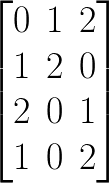# FreBlogg

## Matrix Multiplication | C Programming

09:00 Posted by DurgaSwaroop

In that, I have already shown how Matrix addition and subtraction can be done and asked you to try multiplication themselves. Hopefully you have been able to do that Or atleast tried it out before coming here to see my solution.

For this example, I have taken two matrices as follows.Matrix1 has 3 rows and 4 columns and Matrix2 has 4 rows and 3 columns. And now, the code to multiply these two matrices.
```#include "stdio.h"
main() {

int matrix1 = { {1,2,3,4}, {3,4,1,2}, {4,1,2,3} };
int matrix2 = { {0,1,2}, {1,2,0}, {2,0,1}, {1,0,2} };

// Values from the matrices. No. of columns in matrix1 = No. of rows in matrix2. Matrix Multiplication possible
int rows1 = 3, cols1 = 4, rows2 = 4, cols2 = 3;

// Initialize the resultant matrix with all zeroes
int mult_matrix = {0};

// Loop variables
int i=0, j=0, k=0;

for(i = 0; i < rows1; i++) {
for(j = 0; j < cols2; j++) {
for(k = 0; k < cols1; k++) {
// Since we are initializing with zero, we can add new values to the previous value to get the final value
mult_matrix[i][j] += matrix1[i][k] * matrix2[k][j];
}
}
}

//  Print the new matrix
for(i = 0; i < rows1; i++) {
for(j = 0; j < cols2; j++) {
printf("%d ", mult_matrix[i][j]);
}
printf("\n");
}
}
```

And when you run this program, you get the following output as one would expect:
```12 5 13
8 11 11
8 6 16

Press any key to continue . . .
```
The comments, along with the explanation in the video should be enough for you to understand this code directly.But, do reach out on social media for any queries.